# B-convergence

Jump to: navigation, search

The theory of B-convergence predicts the convergence properties of discretization methods, implicit Runge–Kutta methods in particular, applied to initial value problems for systems of non-linear ordinary differential equations(cf. Runge–Kutta method; Initial conditions; Differential equation, ordinary).

## Problem class and historical background.

Concerning the class of ordinary differential equations considered, the underlying assumption is thatsatisfies a one-sided Lipschitz condition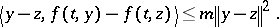If(the so-called one-sided Lipschitz constant of) is of moderate size, the initial value problem is easily proved to be well-conditioned throughout. Of particular interest are stiff problems (cf. also Stiff differential system). Stiffness means that that the underlying ordinary differential equation admits smooth solutions, with moderate derivatives, together with non-smooth ( "transient" ) solutions rapidly converging towards smooth ones. In the stiff case, the conventional Lipschitz constant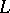ofinevitably becomes large; therefore the classical error bounds for discretization methods (which depend on) are of no use for an appropriate characterization and analysis of methods which are able to efficiently integrate a stiff problem. Thus there is a need for a special convergence theory applicable in the presence of stiffness.

The idea to use(instead of) as the problem-characterizing parameter goes back to [a7], where it was used in the analysis of multi-step methods. The point is that stiffness is often compatible with moderate values of, while. In the same spirit, the concept of B-stability was introduced in [a4], [a5], [a6] in the context of implicit Runge–Kutta methods, and an algebraic criterion on the implicit Runge–Kutta coefficients entailing B-stability was derived ( "algebraic stability in numerical analysisalgebraic stability" ). The notion of-stability enables realistic estimates of the propagation of inevitable perturbations like local discretization errors.

## The concept of B-convergence.

In the convergence theory of implicit Runge–Kutta methods applied to stiff problems, stability is essential but also the analysis of local errors is non-trivial: straightforward estimates are affected by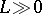and do not reflect reality. For a simple scalar model class, the local error of implicit Runge–Kutta schemes was studied in [a13]. It turned out that the order observed for practically relevant stepsizes is usually reduced compared to smooth, non-stiff situations.

In [a9], [a10], [a11], the convergence properties of implicit Runge–Kutta schemes are studied and the notion of B-convergence is introduced. A B-convergence result is nothing but a realistic global error estimate based on the parameterbut unaffected by. Besides relying on B-stability, the essential point are sharp local error estimates which require a special internal stability property called BS-stability. The latter can be concluded from a certain algebraic condition on the Runge–Kutta coefficients ( "diagonal stability in numerical analysisdiagonal stability" ). Explicit error bounds have been derived for Gauss, Radau-IA and Radau-IIA schemes; the corresponding "B-convergence order" is in accordance with the observations from [a13]. B-convergence results for Lobatto-IIIC schemes are given in [a14].

An overview on the "B-theory" of implicit Runge–Kutta methods is presented in [a8]. Another relevant text is [a12].

## Further developments.

Concerning the relevance of the B-theory for stiff problems, there remains a gap. The point is that for most stiff problems there is a strong discrepancy between the local and the global condition: Neighbouring solutions may locally strongly diverge, such that the problem is locally ill-conditioned. This is a transient effect, leaving the good global condition unaffected. But it inevitably implies that the one-sided Lipschitz constantis strongly positive (like). For details, cf. [a1], where it is shown thatremains moderate only for a restricted class of stiff problems, namely with Jacobians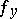that are "almost normal" . In general, however,is large and positive, and the B-convergence bounds based onbecome unrealistically large.

As a consequence, not even linear stiff problems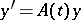are satisfactorily covered. In [a2] the B-theory is extended to semi-linear stiff problems of the form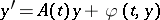, wherehas a smoothly varying eigensystem and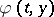is smooth. However, this does not cover a sufficiently large class of non-linear problems. Current work concentrates on a more natural, geometric characterization of stiffness; cf., e.g., [a3].